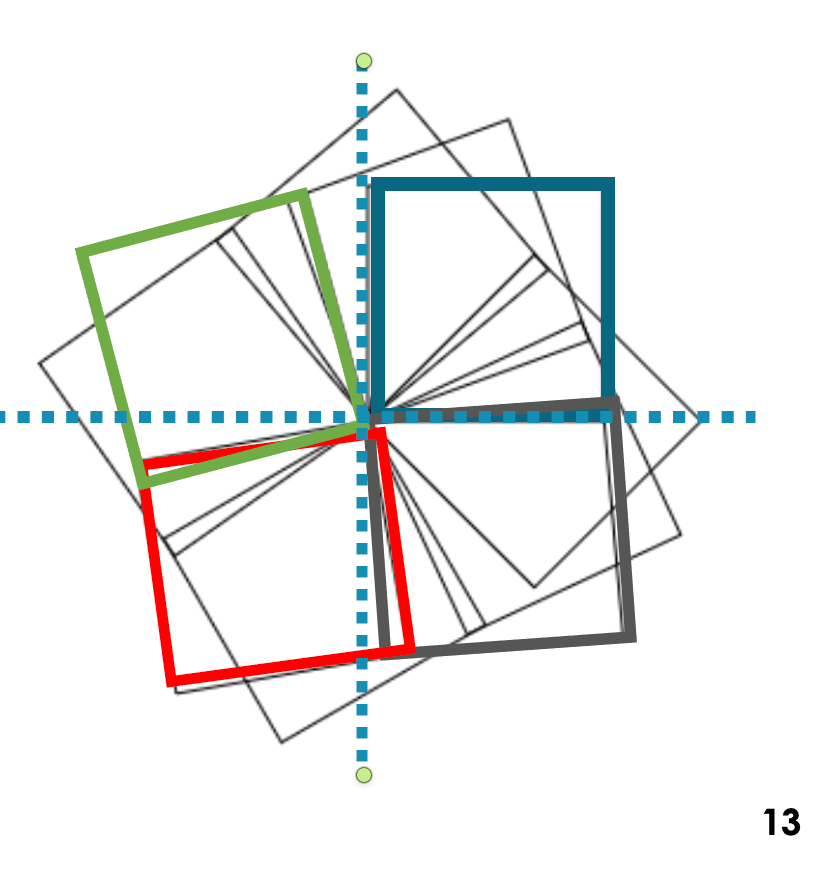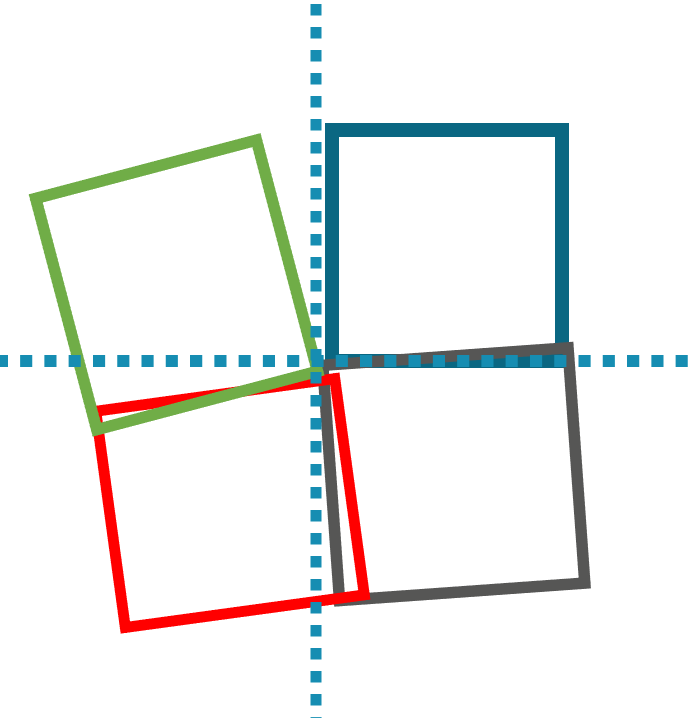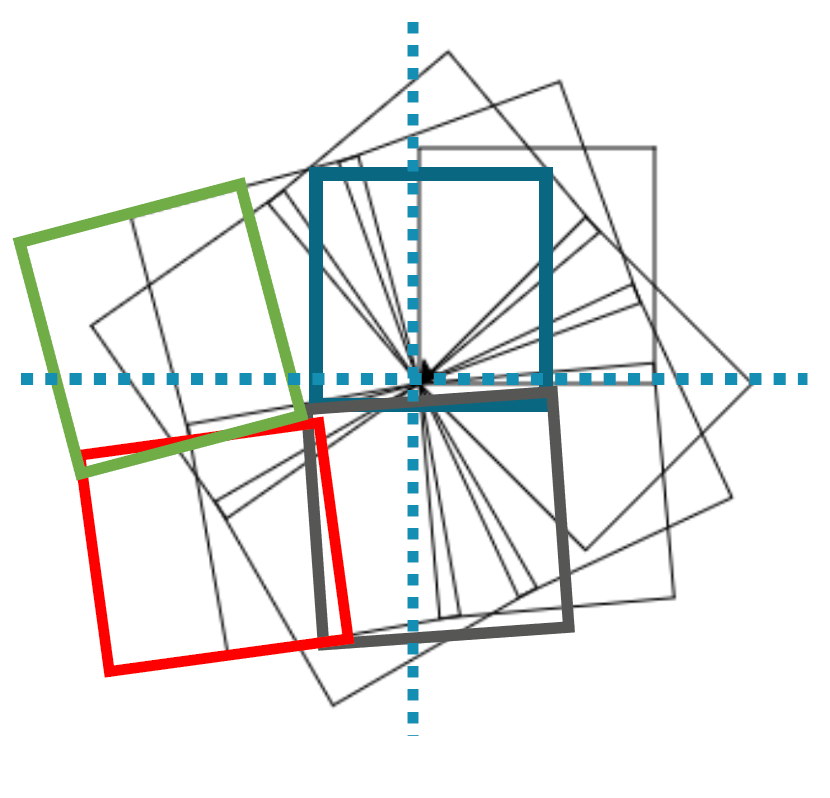# Algorithms

## What is an algorithm

• It is the step by step solution to the given problem.
• It is clear and specific, include all steps and has correct order.
• The algorithm can be expressed in plain spoken language and/or series of calculations, or charts such as flow charts.
• Sometimes there are different solutions or algorithms to solve the same problems.
• Some algorithms are faster and more efficient than others.
• There are many ways to solve the same problem.

### Example-1

List all even numbers from 1 to 1000 2, 4, 6, 8, 10, 12....998, 1000

```
assign 0 to x.      (x = 0)
for number i = 1 to 500   (i = 500)
increase x by 2        	(x = 1000)
Display x
next i

```

### Example-2

Find the maximum number in the following list: 1, 3, 5, 6, 2, 8, 9, 10, 11, 2, 4

```
maxNumber = The first number
for every number in the list
if the number is greater than maxNumber
assign number to the maxNumber
next number
print maxNumber

```

### Example-3

Find all even numbers in the following list: 1, 3, 5, 6, 2, 8, 9, 10, 11, 2, 4

```
for every number in the list
divide the number by 2
get the remainder of the division
if the remainder = 0
the number is even, print it
else
the number is odd, do nothing
next number

```

## Algorithm in computer terms

• An algorithm is a step-by-step process producing a solution for a problem which can be translated into computer code.
• There are four main components of an algorithm: data acquisition, sequence, selection and iteration.
• Writing an algorithm to solve a simple problem may not require all these components.
• An algorithm is usually written in plain (simple) human language, and then translated to a high-level programming language such as Python.

### Example-4

• Assume that you are required to develop an algorithm to draw the shape as below.
•• How can you apply computational thinking to this problem?
• Develop the solution/algorithm, and later use a computer language called Python to draw this shape.

### Solution

• If you divide the shape into four parts, examining each part carefully, you will notice that the shape is made up of squares.
• Each square is rotated slightly by a small angle.
• This process of discovery is called decomposition.
• If you draw one square, then change the angle slightly, draw the next square, and repeat this multiple times, you will get the shape.
•• There are other lines in this shape that are created by having many squares drawn at different angles.
• If you spend too much time at this stage trying to understand how they have been created, you might get confused.
• Focusing on the big picture and finding the most important part of the problem is called abstraction.
• The next step is writing the solution step-by-step using simple language. This is called algorithm.
```         from 1 to the number of boxes
draw a box
change drawing angle by 5 degrees
next box
```